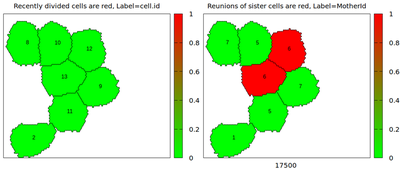# Sister Reunion

##### Persistent Identifier

Use this permanent link to cite or share this Morpheus model:

Track MotherId (cell.id of mother) and compare it between neighboring cells

## Introduction

This example model demonstrates the tracking of the cell.id of any cell’s mother and to compare these MotherIds between neighboring cells upon contact to trigger special behaviors if sister cells meet in a SisterReunion. Such special cell-cell contacts of sister cells are flagged by the cell property SisterReunion $= 1$, otherwise $0$.

## Description

For each cell division, a trigger copies the cell.id of the mother cell to the property MotherId of each daughter cell. In the included movie, the left panel shows cell.id, the right panel MotherId. The plugin NeighborhoodReporter compares local.MotherId == MotherId where local accesses the cell’s own scope when collecting information from the neighborhood. The test returns a $1$ when sisters are in contact, $0$ otherwise. This is updated every timestep (can be adjusted in NeighborhoodReporter) and stored in the Property SisterReunion and used to color cells in the right panel of the movie.Snapshot of moving and dividing cells, daughter cells from the same earlier cell division that later encounter each other again are colored red in the right panel, as indicated by the computed property SisterReunion ($0 =$ green, $1 =$ red)

A follow-up question may be what happens when multiple cells are in contact of which at most two may be sisters. To control the outcome, you may choose a statistical function in the mapping attribute of the NeighborhoodReporter/Output. The statistical function is then applied to all pairwise results of the NeighborhoodReporter with each neighboring cell. In the attached files, I’ve used the mapping attribute maximum to get enough red color into the movie. If you choose minimum then you could flag just those sister reunions that are happening privately. Or you choose scaling="length" and mapping="average" to average over the cell membrane fractions (as opposed to each neighbor having the same weight independent of contact length, which you get with scaling="cell", mapping="average") in contact with neighboring cells (mind the medium).

## Model

Get this model via:

XML Preview

<?xml version='1.0' encoding='UTF-8'?>
<MorpheusModel version="4">
<Description>
<Title>Example-SisterReunion</Title>
<Details>Demonstration for tracking of MotherId and selective behavior for cell-cell interactions of sister cells from an earlier cell division of the joint mother cell. Such special cell-cell contacts of sisters are flagged by the cell property SisterReunion=1, otherwise 0.
Date: April 20, 2020
Author: lutz.brusch@tu-dresden.de

</Details>
</Description>
<Global>
<Variable value="0.0" symbol="c"/>
<Constant value="-1" symbol="MotherId"/>
</Global>
<Space>
<Lattice class="hexagonal">
<Size value="100, 100, 0" symbol="size"/>
<BoundaryConditions>
<Condition type="periodic" boundary="x"/>
<Condition type="periodic" boundary="y"/>
</BoundaryConditions>
<Neighborhood>
<Order>1</Order>
</Neighborhood>
</Lattice>
<SpaceSymbol symbol="space"/>
</Space>
<Time>
<StartTime value="0"/>
<StopTime value="4e4" symbol="stoptime"/>
<TimeSymbol symbol="time"/>
</Time>
<CellTypes>
<CellType name="cells" class="biological">
<Property value="0" symbol="MyId"/>
<Property value="0" symbol="MotherId"/>
<Property value="0" symbol="SisterReunion"/>
<NeighborhoodReporter time-step="1" name="Test Sister Reunion">
<Input scaling="cell" value="local.MotherId==MotherId"/>
<Output symbol-ref="SisterReunion" mapping="maximum"/>
</NeighborhoodReporter>
<Property value="1e-4" symbol="p" name="proliferation rate"/>
<Property value="0" symbol="d" name="divisions"/>
<Property value="0" symbol="c" name="color"/>
<VolumeConstraint strength="1" target="500"/>
<SurfaceConstraint strength="1" target="0.9" mode="aspherity"/>
<System time-step="1.0" solver="euler">
<Rule symbol-ref="c">
<Expression>if( c > 0, c-1, 0)</Expression>
</Rule>
</System>
<CellDivision division-plane="random" orientation="1.0, 0.0, 0.0">
<Condition>rand_uni(0,1) &lt; p</Condition>
<Triggers>
<Rule symbol-ref="d">
<Expression>d+1</Expression>
</Rule>
<Rule symbol-ref="c" name="color after division">
<Expression>1000</Expression>
</Rule>
<Rule symbol-ref="MotherId">
<Expression>MyId</Expression>
</Rule>
</Triggers>
</CellDivision>
<DirectedMotion strength="0.1" direction="mod(cell.id,2)-0.5, 0.0, 0.0" name="JustForFunToSeparateSistersAfterDivision"/>
<Event time-step="10" trigger="when true" name="UpdateMyIdAfterInheritance">
<Condition>1</Condition>
<Rule symbol-ref="MyId">
<Expression>cell.id</Expression>
</Rule>
</Event>
</CellType>
</CellTypes>
<CPM>
<Interaction default="0">
<Contact value="-4" type2="cells" type1="cells"/>
</Interaction>
<MonteCarloSampler stepper="edgelist">
<MCSDuration value="1.0"/>
<Neighborhood>
<Order>1</Order>
</Neighborhood>
<MetropolisKinetics yield="0.1" temperature="3"/>
</MonteCarloSampler>
<ShapeSurface scaling="norm">
<Neighborhood>
<Order>3</Order>
</Neighborhood>
</ShapeSurface>
</CPM>
<CellPopulations>
<Population size="1" type="cells">
<InitCircle mode="regular" number-of-cells="1">
<Dimensions center="50, 50, 0" radius="5"/>
</InitCircle>
</Population>
</CellPopulations>
<Analysis>
<Gnuplotter time-step="100" decorate="true">
<Terminal name="png"/>
<Plot title="Recently divided cells are red, Label=cell.id">
<Cells value="c" max="1" min="0.0">
<ColorMap>
<Color value="1" color="red"/>
<Color value="0.0" color="green"/>
</ColorMap>
</Cells>
<CellLabels value="cell.id" fontsize="12"/>
</Plot>
<Plot title="Reunions of sister cells are red, Label=MotherId">
<Cells value="SisterReunion" max="1" min="0.0">
<ColorMap>
<Color value="1" color="red"/>
<Color value="0.0" color="green"/>
</ColorMap>
</Cells>
<CellLabels value="MotherId" fontsize="12"/>
</Plot>
</Gnuplotter>
<Logger time-step="100">
<Input>
<Symbol symbol-ref="celltype.cells.size"/>
</Input>
<Output>
<TextOutput/>
</Output>
<Plots>
<Plot time-step="10000" log-commands="true">
<Style style="linespoints" point-size="0.5" grid="true"/>
<Terminal terminal="png"/>
<X-axis maximum="stoptime" minimum="0">
<Symbol symbol-ref="time"/>
</X-axis>
<Y-axis maximum="100" minimum="0">
<Symbol symbol-ref="celltype.cells.size"/>
</Y-axis>
</Plot>
</Plots>
</Logger>
<DependencyGraph reduced="false" format="svg"/>
</Analysis>
</MorpheusModel>


The simulation is visualized in this movie linked below the figure: SisterReunionMovie.mp4.